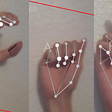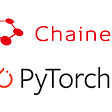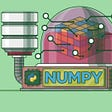# An introduction to Random Forest using Tesla stock prices

In this post, we will aim at having a deeper understanding of Random Forest by taking OHLC (open-high-low-close) data for Tesla stock for 5 year period. We will keep unrelated discussion at a minimum and try to focus on everything that is related to our understanding of Random Forest for this post. It would be helpful if you are familiar with concepts such as mean squared error and R-Squared statistics but it’s not necessary to understand this post.

We will experiment with few features in our dataset to predict the adjusted closing price of Tesla stock for a particular day. These predictions aren’t necessarily good but are only used for illustrative purposes, since the intention of this post is to learn about Random Forest.

An accompanying Jupyter notebook and dataset is provided here.

Code is based on scikit-learn and fastai 0.7.0 packages.

## Decision Trees

We will start by keeping things as simple as possible and later build on our understanding from this tutorial. For now, we will just say that Random Forest is composed of multiple Decision Trees. We will ignore the “Random” and “Forest” part of this equation for now.

A single Decision Tree looks something like the following (ignore the numbers in the nodes for now):

As the name suggests, decisions are made by splitting data into groups that are homogenous within themselves but heterogenous with others. A split is based on some criteria (score) that achieves the best possible division of data in a way that optimizes for that criteria. For example, criteria can be a decision split that reduces the “mean squared error”. We call this criteria, information gain which basically tells us how much our criteria improves as we keep on making those splits. Two splits at each node generally suffice because the tree can have an infinite depth (as long as some stopping condition is reached) so there is no need to make more than two splits at each node.

We will start by first constructing and visualizing a very simple single Decision Tree using scikit-learn and some code from the fastai library.

To keep things simple, we will only use a single feature for now, the Adj. Open price.

`TOTAL = df.count() N_VALID = 90 # Three months TRAIN = TOTAL - N_VALID df_small = df features = ['Adj. Open'] X_df = df_small[features] y_df = df_small['y']  X_train, X_val = X_df[:TRAIN], X_df[TRAIN:] y_train, y_val = y_df[:TRAIN], y_df[TRAIN:]model = RandomForestRegressor(n_estimators=1, max_depth=2, bootstrap=False, min_samples_leaf=1)model.fit(X_train, y_train)draw_tree(model.estimators_, X_train, precision=2)`

Each node in this case predicts the `value(y)` to be the mean of samples in that node. You can test this out by yourself for the first node as follows.

`y_train.mean()`

This outputs 159.05220496338478, the same as the value in first node of the image

In the image above, `samples` is the number of rows used and `mse` is the mean squared error.

At depth = 0, the best prediction that it can make for Adj. Open with the available information at that node is that the predicted y is the average, which is only fair as it hasn’t done much at that point.

At depth = 1, the model has found a split at Adj. Open = 128.73 such that any value less than or equal to 128.73 goes into the lower (False) node and everything else goes to the upper (True) node.

For each individual split,

• it iterates through all the rows (since bootstrap is disabled)
• at each iteration, it partitions `X_train` into left and right hand side (lhs, rhs) and calculates some score and stores it
• after it has gone through all iterations, it selects the partition that optimizes the criteria (gives a lower mse in this case), i.e. decides on the split

We deliberately skipped explaining parameters in `RandomForestRegressor` so let’s discuss this here before we dive further.

`RandomForestRegressor(n_estimators=1, max_depth=2, bootstrap=False, min_samples_leaf=1)`

`n_estimators`: Specifies the number of decision trees we want to use. Since we are ignoring the "Random" and "Forest" part of the equation, we want to limit it to a single Decision Tree. Remember that Random Forest is just an ensemble of Decision Trees.

`max_depth`: The depth till which we want to go per decision tree. To keep things simple and easily visualizable, we have kept it to 2 for now.

`bootstrap`: Bootstrap is a technique to pick random samples with replacement. We have disabled it for better explainability.

`min_samples_leaf`: This is the minimum number of samples required before considering a tree as leaf and stop splitting. In case where `max_depth` is set, it obviously takes precedence over `min_samples_leaf`.

Now, what happens when there are more than one feature?

In that case, it repeats the aforementioned procedure for all the features and selects the one that gives the lowest mse. This is demonstrated below for the same dataset.

## RMSE and R-Squared for model with single feature

`print_score(model)`

rmse train 17.384888739353396
rmse val 19.506417396221188
r^2 train 0.9584178577580958
r^2 val 0.5602690120041153

## RMSE and R-Squared for model with two features

`df_small = dffeatures = ['Adj. Open', 'Adj. Low']X_df = df_small[features]y_df = df_small['y']X_train, X_val = X_df[:TRAIN], X_df[TRAIN:]y_train, y_val = y_df[:TRAIN], y_df[TRAIN:]model = RandomForestRegressor(n_estimators=1, max_depth=2, bootstrap=False, min_samples_leaf=1)model.fit(X_train, y_train)draw_tree(model.estimators_, X_train, precision=2)print_score(model)`

rmse train 16.976452987498227
rmse val 19.357544984072113
r^2 train 0.9603487449090349
r^2 val 0.5669554273097887

Notice how the model decided to split on Adj. Low instead of Adj. Open as opposed to when we forced it to only use Adj. Open for predictions in the previous case. We can also see why it did that. In the second model RMSE, obtained is lower and R-squared is higher for both train and validation datasets. Pretty cool stuff!

Note that it is not necessary that deeper nodes will split on the same feature as the first node. We will notice this later in the post.

Now let’s toss in another feature and see how it performs.

`df_small = dffeatures = ['Adj. Open', 'Adj. Low', 'Adj. Close']X_df = df_small[features]y_df = df_small['y']X_train, X_val = X_df[:TRAIN], X_df[TRAIN:]y_train, y_val = y_df[:TRAIN], y_df[TRAIN:]model = RandomForestRegressor(n_estimators=1, max_depth=2, bootstrap=False, min_samples_leaf=1)model.fit(X_train, y_train)draw_tree(model.estimators_, X_train, precision=2)print_score(model)`

rmse train 16.81048890819006
rmse val 19.268735911850047
r^2 train 0.9611202272940632
r^2 val 0.5709197798942935

Third model seems to be the best one so far but there is a catch! Adj. Close is the same as y, the value we are trying to predict and the model naturally comes to the conclusion that Adj. Close is the best feature to predict y. Wow!

But this is just mentioned to prove a point. There is no point in using y to predict y!

So now that we have a basic understanding of Decision Trees, let’s try to properly fit a Decision Tree model with just two features, Adj. Open and Adj. Low.

`df_small = dffeatures = ['Adj. Open', 'Adj. Low']X_df = df_small[features]y_df = df_small['y']X_train, X_val = X_df[:TRAIN], X_df[TRAIN:]y_train, y_val = y_df[:TRAIN], y_df[TRAIN:]model = RandomForestRegressor(n_estimators=1, bootstrap=True, min_samples_leaf=25)model.fit(X_train, y_train)draw_tree(model.estimators_, X_train, precision=2)print_score(model)`

rmse train 5.272029026362083
rmse val 5.330317829943744
r^2 train 0.9961759935699128
r^2 val 0.9671649065646027

`model.estimators_.get_depth()`

Depth = 6

We have managed to vastly improve R-Squared for both train and validation set. Since there was no limit on the depth, the final tree that was build turned out having a depth of 6 with at least a minimum of 25 samples in its leaf nodes. To go further deep, it has to at least have 25 samples in the leaf, or else it stops. We have also enabled bootstrapping to avoid overfitting.

Also, notice that it used both features and split each node by selecting the one that was most optimal.

We can begin optimizing our current model by using Random Forest and see if we can further improve on RMSE and R-Squared values.

## Random Forest

Random Forest is constructed using a technique called Bagging which works like the illustration below.

• For each of the decision tree, pick a random subset of training data and fit a Decision Tree on that. Do that as many times as the number of trees specified for a forest.
• Now, each model is somewhat predictive for a subset of data. Since the subset was randomly chosen, errors for each of these models shouldn’t be correlated and this is important.
• If we take the average of these different models, we are effectively merging information contained in all of these models which represents the full dataset.

This technique for ensembling Decision Trees is what is called Random Forest.

Let’s train a Random Forest model now with the same two features that we used before. Note that `n_estimators` in scikit-learn are Decision Trees and we will use 40 of them.

`df_small = dffeatures = ['Adj. Open', 'Adj. Low']X_df = df_small[features]y_df = df_small['y']X_train, X_val = X_df[:TRAIN], X_df[TRAIN:]y_train, y_val = y_df[:TRAIN], y_df[TRAIN:]model = RandomForestRegressor(n_estimators=40, bootstrap=True, min_samples_leaf=25)model.fit(X_train, y_train)draw_tree(model.estimators_, X_train, precision=2)print_score(model)`

rmse train 4.397823614729115
rmse val 4.201441978163789
r^2 train 0.9973390381853801
r^2 val 0.9796000648754558

We can see a slight improvement in RMSE and R-Squared for both datasets which is what we want. Our model seems to be working pretty well.

Remember that we mentioned that the average of all these trees indicates our prediction. This holds true as follows.

For each of the Decision Trees, we get the predicted values. We then calculate the mean of predictions from all trees for a single datapoint. That mean value turns out to be very close to the y that we were trying to predict and is the same as what will be returned by the collective prediction of all the 40 Decision Trees.

`preds = np.stack([t.predict(X_val) for t in model.estimators_])print(f"Trees: {len(preds[:,0])},", f"Mean of 0th row for prediction from all trees: {np.mean(preds[:,0])},", f"Actual y: {y_val}")`

Trees: 40, Mean of 0th row for prediction from all trees: 188.32322750181893, Actual y: 185.02

Prediction from the Random Forest model is the same as the mean calculated above by explicitly taking an average.

`model.predict(X_val)`

Output: 188.32322750181896

Now, there are different hyper parameters that we can experiment with to tune our model. We are not going to cover that in this blog post but just to give some taste, the following plot builds up an understanding for that.

In the following, we plot R-Squared against different number of Decision Trees used. As we increase the number of trees, we improve R-Squared (closer to 1 is better) and hence we are better able to tune our model.

However, somewhere around 15 trees, we stop seeing any further improvement in R-Squared statistic and the line tends to flatten out. This tells us that approximately 15 Decision Trees are as good as 40 in our example.

`plt.plot([metrics.r2_score(y_val, np.mean(preds[:i+1], axis=0)) for i in range(40)]);`

## Conclusion

In this blog post, we started with the very basics of a Decision Tree and used that to build our understanding of the Random Forest.

We used a pre-cleaned dataset that was already feature-engineered so there wasn’t any discussion around that. And although, we trained a pretty decent model using just two features, we can do even better by using more features and more hyper parameter tuning.

Finally, this blog post wouldn’t have been made possible without my learnings from Jeremy Howard’s fastai courses (http://course18.fast.ai/ml.html).

--

--

--

## More from Uncanny Recursions

A software engineer’s journey around tech and product

## Introduction to Hyperspectral Remote Sensing -PART I## Item-Based Collaborative Filtering## Using Neural Networks to Improve Waste Management, One Propagation at a Time.## How to Create your own Sign Language Translation App by extending SigNN## Pump Failure Detection App-Application based on IIoT## Yes, it’s worthy: Einstein summation notation applied to Machine Learning.## Using MobileNetV2 to Face Mask Images Detect in Python## Migration from Chainer to PyTorch## Rafay Aleem

Data Engineer at Faire. Based in Toronto. Music aficionado who likes playing guitar and is an Eric Clapton fan.

## YouTube Video Review: Learn Data Science by Doing Kaggle Competitions: Connect X## City Happiness Level EDA & Regression Model## How does Mahout work?## Essential Libraries To Have In Your Tool For Data Science And ML — Series #1 — NumPy